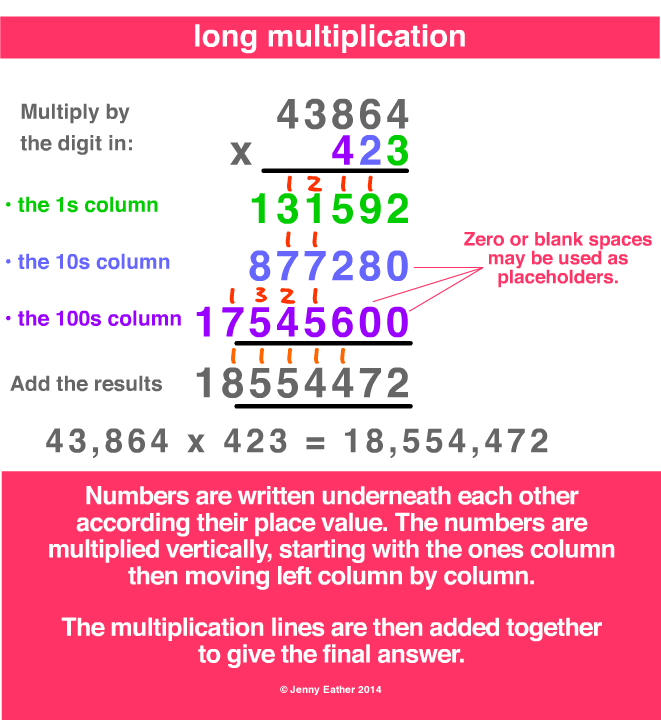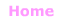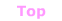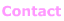Aa Bb Cc Dd Ee Ff Gg Hh Ii Jj Kk Ll Mm Nn Oo Pp Qq Rr Ss Tt Uu Vv Ww Xx Yy Zz

Aa

arithmetic

• area of mathematics that includes:
- addition, subtraction, multiplication and division, of
- whole numbers, decimals and fractions.

EXAMPLE: long multiplicationcommon operations

Note: Due to the commutative property of addition,
use of the word ”augend" for the first term in addition is rarely used today,
and both terms are usually called addends.

Subtraction (−)
minuend − subtrahend = difference

Multiplication (×)
multiplicand × multiplier = product

Division (÷)
dividend ÷ divisor = quotient© Jenny Eather 2014. All rights reserved.